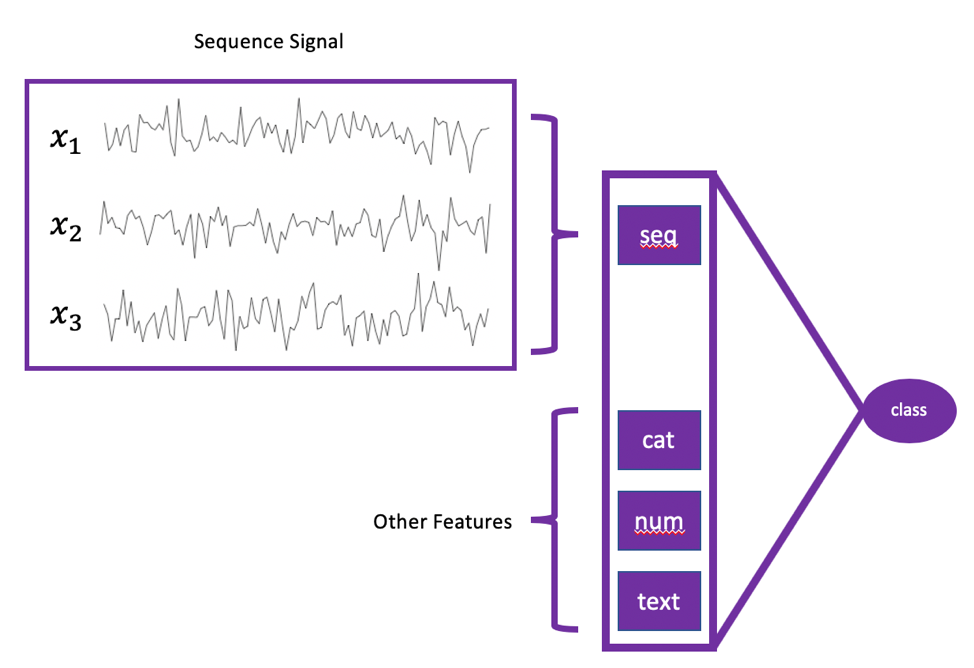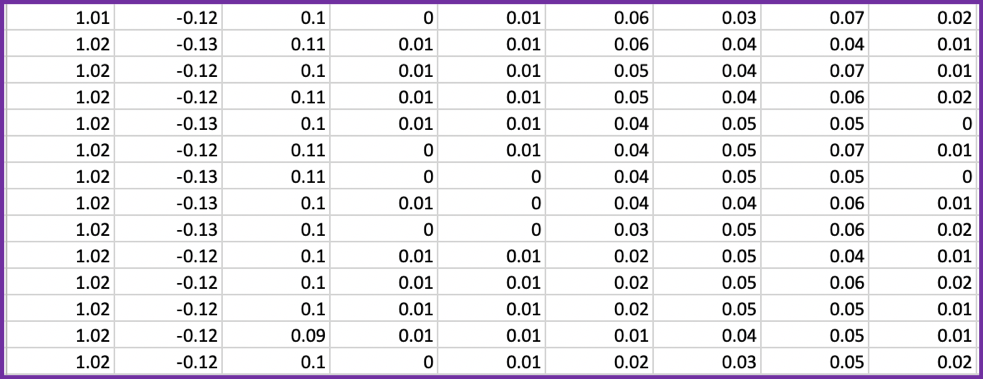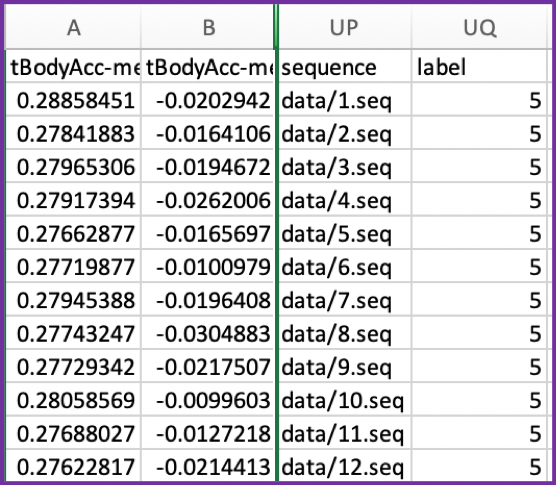# Time series classification

Time series classification is an important branch in time series analysis. The sample is classified based on historical observation sequence signals. Many practical applications fall into this category. For example, detecting diseases by analyzing ECG or EEG sequences, human behavior recognition from wearable IoT sensory data, preventative maintenance on various hardware parts, such as automobile, aircraft, computer server, and factory machinery.

This tutorial will guide you through steps to build AI models to classify time series sequences.

The OneClick.ai platform supports mixed types of inputs: sequence, numerical, categorical, images, text or any combination of those mentioned. The diagram below is one example of valid data.Based on the above data format, users need to prepare two types of files to support time series classification:

1. Sequence files: save the time sequences of each sample as a separate file, which does not need to have a header. It is recommended to separate the different sequences ​​with commas within the same file. For example, if a machine has 3 sensors generating sequence data and the length of each is 128 steps, the data will be stored in a file of 128 rows and three columns, each row representing one observation of the three sensors’ out in a timestep.
2. Label file: other numerical, categorical, text or image features and real targets are placed in this csv/txt file. Furthermore, an additional column needs to be added to the csv to represent the relative path to the sequence files above.

Let us use a UCI open dataset as an example. This dataset contains sensor measurements from 30 volunteers in six different activities (walking, going down the stairs, climbing stairs, sitting, standing, lying). The measurement data was obtained from the accelerometers of the smartphone in the waist of the volunteers and the nine observations of the gyroscope. The observations were sampled at a frequency of 50 Hz. In addition, the data provider generated 561 statistical values ​​based on 9 sequences. A more detailed description of the data can be found in the link above.

Based on the raw data, we need to prepare two files:

1. A folder contains the measured values ​​of the sequences. Each file contains 128 rows, 9 columns and must have ‘.seq’ as the file extension. OneClick.ai uses this extension to recognize the sequence file.
2. A csv file contains 561 statistical values, relative paths to the sequence files, and the ground truth value for the prediction target.

Here are the steps to prepare this dataset:

• Unzip it, go to the folder, create two folders named “data” and “test_data”.
• Run the following script to generate the required input data to run on the platform.
import pandas as pd
import numpy as np

return dataframe.values

for name in filenames:
# stack group so that features are the 3rd dimension

filepath = prefix + group + '/Inertial Signals/'
# load all 9 files as a single array
filenames = list()
# total acceleration
filenames += ['total_acc_x_'+group+'.txt', 'total_acc_y_'+group+'.txt', 'total_acc_z_'+group+'.txt']
# body acceleration
filenames += ['body_acc_x_'+group+'.txt', 'body_acc_y_'+group+'.txt', 'body_acc_z_'+group+'.txt']
# body gyroscope
filenames += ['body_gyro_x_'+group+'.txt', 'body_gyro_y_'+group+'.txt', 'body_gyro_z_'+group+'.txt']
y = load_file(prefix + group + '/y_'+group+'.txt')
return X, y

def save_sequence_file(folder, X_seq):
# X_seq is [n_samples, time_steps, n_signal] array
sequence = []
for i in range(train_X.shape):
filename = folder + '/' + str(i+1) + '.seq'
sequence.append(filename)
x_seq = train_X[i, :, :]
np.savetxt(filename, x_seq, delimiter=&amp;amp;amp;amp;amp;amp;quot;,&amp;amp;amp;amp;amp;amp;quot;, fmt='%1.2f')

return sequence

X_train = pd.DataFrame(X_train, columns=features['feature_names'].values)
sequence = save_sequence_file('data', train_X)
# save train to dataframe
train = X_train.copy()
train['sequence'] = sequence
train['label'] = train_y[:, 0]
train.to_csv(&amp;amp;amp;amp;amp;amp;quot;human_activities_recognition_train.csv&amp;amp;amp;amp;amp;amp;quot;, index=False)

# process test
X_test = pd.DataFrame(X_test, columns=features['feature_names'].values)
test_sequence = save_sequence_file('test_data', test_X)
test = X_test.copy()
test['sequence'] = test_sequence
test['label'] = test_y[:, 0]
test.to_csv(&amp;amp;amp;amp;amp;amp;quot;human_activities_recognition_test.csv&amp;amp;amp;amp;amp;amp;quot;, index=False)


• Select “human_activities_recognition_train.csv” and “data” folder, right click to compress them into a file.
• Select “human_activities_recognition_test.csv” and “test_data” folder, right click to compress them into a file.
• Upload the first file to OneClick.ai to train the model and later upload the latter one to test the model performance.

Screenshot of a file containing 9 sequences:Screenshot of the file containing other features, sequence file path, and labels:Note:

• The sequence file currently only supports numerical.
• In case that each sequence file has a different length, OneClick.ai can automatically handle it without users doing any manual processing.